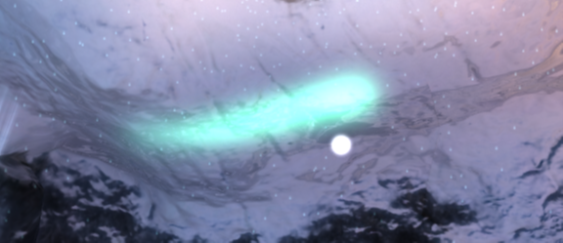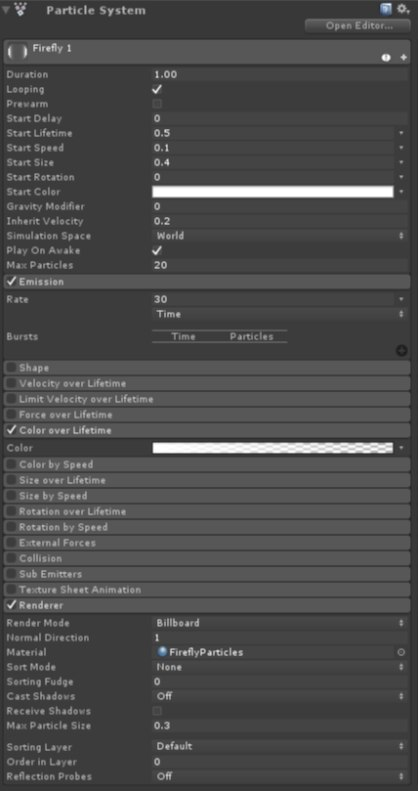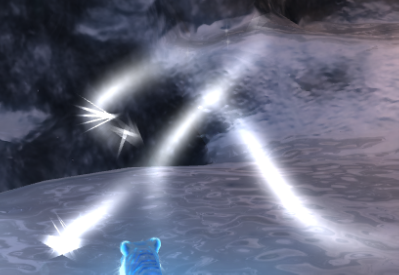## Fireflies

This section of the guide shows you how to implement fireflies into your Unity programs. Fireflies are bright flying insects that are used in the Ice Cave demo to add more dynamism.

The fireflies are composed of the following components:

• A prefab object that is instantiated at runtime
• A box collider that is used to limit the area the fireflies can fly around in

These two components are combined in a C# script that manages the movement of the fireflies and defines the path that they follow.

The following image shows a firefly:The firefly prefab uses the Unity standard particle system to generate the trail of the firefly.

The following image shows the Unity Particle System settings that the fireflies use:Depending on the effect that you want to achieve, you can use a Unity Trail Renderer to provide a more continuous look.

The Trail Renderer generates many triangles for each trail. You can change the number of triangles by modifying the Trail Renderer setting Min Vertex Distance. However, if the source is moved too fast, a high value can cause a jerky movement of the trail.

The Min Vertex Distance option defines the minimum distance between the vertices that form the trail. A high number is okay for straight trails, but it does not look smooth for curved trails.

The trail that is generated always faces the camera. Therefore, any abrupt movement of the source can cause the trail to overlap with itself. This causes artifacts that are generated by the blending of the triangles that form the trail.

The following image shows artifacts caused by an overlapping trail:The final component to add to the prefab is a point light that casts light in the scene while it is moving.

#### The firefly generator prefab

The firefly generator prefab manages the creation of the fireflies and updates them in each frame. The firefly generator prefab is composed of a C# script for the update, and a box collider that encloses the volume that each firefly can move in.

The script takes the number of fireflies to be generated as a parameter, and the prefab to instantiate the firefly object. This is because the movement of the fireflies is random within the bounding box, it limits changes to a specific angle, away from the direction that the firefly is moving. This ensures that the firefly does not make sudden changes of direction.

To generate a random movement, a piecewise cubic Hermite interpolation is used to create the control points. The Hermite interpolation provides a smooth continuous function that behaves correctly even when different paths are connected. The first derivative at the end points are also continuous, so that there are no sudden velocity changes.

This interpolation requires one control point each for the start and the end, and two tangents for each of the control points. This is because the control point and the tangents are generated randomly, the script stores three control points and two tangents. The interpolation uses the position of the first and second control points to define the first point tangent. The second and third control points are used to define the tangent of the second control point.

• An initial position
• An initial direction that uses the Unity function `Random.onUnitSphere`

The following code shows how the control points are initialized:

```_fireflySpline[i*_controlPoints] = initialPosition;
Vector3 randDirection = Random.onUnitSphere;
_fireflySpline[i*_controlPoints+1] = initialPosition + randDirection;
_fireflySpline[i*_controlPoints+2] = initialPosition + randDirection * 2.0f;
```

The initial control points lie on a straight line. The tangents are generated from the following control points:

```//The tangent for the first point is in the same direction as the initial direction vector
_fireflyTangents[i*_controlPoints] = randDirection;
//This code computes the tangent from the control point positions. It is shown here for
// reference because it can be set to randDirection at initialization.
_fireflyTangents[i*_controlPoints+1] = (_fireflySpline[i*_controlPoints+2] -
_fireflySpline[i*_controlPoints+1])/2 + (_fireflySpline[i*_controlPoints+1] -
_fireflySpline[i*_controlPoints])/2;
```

Note: To complete a firefly initialization, you must set the color of the firefly and the duration of the current path interval.

On each frame, the script updates the position of each firefly that uses the following code to compute the Hermite interpolation:

```// t is the parameter that defines where in the curve the firefly is placed. It represents
// the ratio of the time the firefly has traveled along the path to the total time.
//Hermite interpolation parameters
Vector3 A = _fireflySpline[i*_controlPoints];
Vector3 B = _fireflySpline[i*_controlPoints+1];
float h00 = 2*Mathf.Pow(t,3) - 3*Mathf.Pow(t,2) + 1;
float h10 = Mathf.Pow(t,3) - 2*Mathf.Pow(t,2) + t;
float h01 = -2*Mathf.Pow(t,3) + 3*Mathf.Pow(t,2);
float h11 = Mathf.Pow(t,3) - Mathf.Pow(t,2);
//Firefly updated position
_fireflyObjects[i].transform.position = h00 * A + h10 * _fireflyTangents[i*_controlPoints] + h01 * B + h11 * _fireflyTangents[i*_controlPoints+1];
```

If the firefly completed the whole piece of randomly generated path, the script creates a new random piece that starts from the end of the current one. This is shown in the following code:

```// t > 1.0 indicates the end of the current path
if (t >= 1.0)
{
//Update the new position
//Shift the second point to the first as well as the tangent
_fireflySpline[i*_controlPoints] = _fireflySpline[i*_controlPoints+1];
_fireflyTangents[i*_controlPoints] = _fireflyTangents[i*_controlPoints+1];
//Shift the third point to the second, this point doesn't have a tangent
_fireflySpline[i*_controlPoints+1] = _fireflySpline[i*_controlPoints+2];
//Get new random control point within a certain angle from the current fly direction
_fireflySpline[i*_controlPoints+2] = GetNewRandomControlPoint();
//Compute the tangent for the central point
_fireflyTangents[i*_controlPoints+1] = (_fireflySpline[i*_controlPoints+2] -
_fireflySpline[i*_controlPoints+1])/2 + (_fireflySpline[i*_controlPoints+1] -
_fireflySpline[i*_controlPoints])/2;
//Set how long should take to navigate this part of path